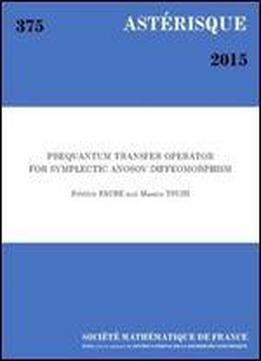# Prequantum Transfer Operator For Symplectic Anosov Diffeomorphism by Faure / 2018 / English / PDF

We define the prequantization of a symplectic Anosov diffeomorphism f:MM as a U(1) extension of the diffeomorphism f preserving a connection related to the symplectic structure on M. We study the spectral properties of the associated transfer operator with a given potential VC^(M), called prequantum transfer operator. This is a model of transfer operators for geodesic flows on negatively curved manifolds (or contact Anosov flows). We restrict the prequantum transfer operator to the N-th Fourier mode with respect to the U(1) action and investigate the spectral property in the limit N, regarding the transfer operator as a Fourier integral operator and using semi-classical analysis. In the main result, under some pinching conditions, we show a ``band structure'' of the spectrum, that is, the spectrum is contained in a few separated annuli and a disk concentric at the origin. We show that, with the special (Hlder continuous) potential V_0=12Df_E_u, where E_u is the unstable subspace, the outermost annulus is the unit circle and separated from the other parts. For this, we use an extension of the transfer operator to the Grassmanian bundle. Using Atiyah-Bott trace formula, we establish the Gutzwiller trace formula with exponentially small reminder for large time. We show also that, for a potential V such that the outermost annulus is separated from the other parts, most of the eigenvalues in the outermost annulus concentrate on a circle of radius (V-V_0) where . denotes the spatial average on M. The number of the eigenvalues in the outermost annulus satisfies a Weyl law, that is, N^dVol(M) in the leading order with d=12dimM. We develop a semiclassical calculus associated to the prequantum operator by defining quantization of observables Op_N() as the spectral projection of multiplication operator by to this outer annulus. We obtain that the semiclassical Egorov formula of quantum transport is exact. The correlation functions defined by the classical transfer operator are governed for large time by the restriction to the outer annulus that we call the quantum operator. We interpret these results from a physical point of view as the emergence of quantum dynamics in the classical correlation functions for large time. We compare these results with standard quantization (geometric quantization) in quantum chaos.

views: 177